Fourier Series Demo

### Fourier Series Demo

#### Interactive Fourier Series Coefficient Demo

The scope is clickable & draggable - interactive demo is here. The Fourier Series Coefficient plots are draggable as well (drag the marker).

Your browser does not support the HTML5 canvas tag.
Your browser does not support the HTML5 canvas tag.
Your browser does not support the HTML5 canvas tag.

Control Panel

Signal Type: Square
Triangle
Ramp (Sawtooth)
Full-wave Rectified Sine
Full-wave Rectified Cos
Half-wave Rectified Sine
Half-wave Rectified Cosine
Settings: # of Fourier coefficients:
1
Phase Shift [%]:
0 %
Your browser does not support the HTML5 canvas tag.

This code calculates Fourier Series Coefficients of the selected time-domain signal.

% Control Systems Academy Example 18
%
% This example calculates the Fourier Series coefficients from various
% waveforms. Signals are reconstructed using the original (not modified by
%
% 6/30/2017

clc; clear;

Ts = 0.1e-3; % sampling time
T = 0.2; % signal period

t = linspace(0,1,1/Ts+1); % form a time vector
x = zeros(1, length(t)); % form a zero vector to hold the selected waveform
y = zeros(1, length(t)); % form a zero vector to hold the selected waveform

which_wave = 'half_rectified_cosine'

num_of_coeffs = 5;

phase_shift = 0.0

% Make the desired wave
for k = 1:length(t),
t_mod = t(k) + T + phase_shift*T;
if strcmp(which_wave,'square')
if(mod(t_mod,T) <= (T/2))
x(k) = 1;
else
x(k) = - 1;
end
elseif strcmp(which_wave,'triangle')
x(k) = 4/T * (abs(mod(t_mod, T) - T/2) - T/4);
elseif strcmp(which_wave,'ramp')
if(mod(t_mod,T) <= (T/2))
x(k) = mod(2*t_mod,T)/T;
else
x(k) = mod(2*t_mod,T)/T - 1;
end
elseif strcmp(which_wave,'full_rectified_sine')
x(k) = abs(sin(2*pi*t_mod/T));
elseif strcmp(which_wave,'full_rectified_cosine')
x(k) = abs(cos(2*pi*t_mod/T));
elseif strcmp(which_wave,'half_rectified_sine')
x(k) = sin(2*pi*t_mod/T);
if(x(k) < 0), x(k) = 0; end
elseif strcmp(which_wave,'half_rectified_cosine')
x(k) = cos(2*pi*t_mod/T);
if(x(k) < 0), x(k) = 0; end
end
end

% Determine Fourier coefficients

a = [];
b = [];

a0 = 2*sum(x)/length(y);
c0 = a0 / 2;

for n = 1:num_of_coeffs,

a(n) = 2/T*sum(x .* cos(2*pi*n*t / T)) / (length(y) / T);
b(n) = 2/T*sum(x .* sin(2*pi*n*t / T)) / (length(y) / T);
c(n) = a(n)/2-j*b(n)/2;

end

% Form the complete c(n) vector
f = zeros(1,31);
for n = 1:num_of_coeffs
f(16+n) = c(n);
f(16-n) = conj(c(n));
end

f(16) = c0;

% Reconstruct the signal

y = y + c0;

for n = 1:num_of_coeffs,

y = y + a(n) * cos(2*pi*n*t/T) + b(n) * sin(2*pi*n*t/T);

end

subplot(311)
plot(t,x,t,y)
grid on
xlabel('time [s]')
ylabel('Amplitude')
subplot(312)
stem(-15:15,abs(f))
grid on
xlabel('c[n]')
ylabel('Magnitude')
subplot(313)
stem(-15:15,angle(f))
grid on
xlabel('c[n]')

% no more

This code reconstruct the time domain signal from the Fourier Series coefficients modified using the stem plots above.

% Control Systems Academy Example 18
%
% This example reconstructs the signal using the modifier Fourier series
% coefficients
%
% 6/27/2017

clc; clear;

Ts = 0.1e-3; % sampling time
T = 0.2; % signal period

t = linspace(0,1,1/Ts+1); % form a time vector
x = zeros(1, length(t)); % form a zero vector to hold the selected waveform
y = zeros(1, length(t)); % form a zero vector to hold the selected waveform

a0 = 0;

a(1) = 0;

a(2) = 0;

a(3) = 0;

a(4) = 0;

a(5) = 0;

a(6) = 0;

a(7) = 0;

a(8) = 0;

a(9) = 0;

a(10) = 0;

a(11) = 0;

a(12) = 0;

a(13) = 0;

a(14) = 0;

a(15) = 0;

b(1) = 0;

b(2) = 0;

b(3) = 0;

b(4) = 0;

b(5) = 0;

b(6) = 0;

b(7) = 0;

b(8) = 0;

b(9) = 0;

b(10) = 0;

b(11) = 0;

b(12) = 0;

b(13) = 0;

b(14) = 0;

b(15) = 0;

num_of_coeffs = 5;

c0 = a0 / 2;

for n = 1:num_of_coeffs,

c(n) = a(n)/2-j*b(n)/2;

end

% Form the complete c(n) vector
f = zeros(1,31);
for n = 1:num_of_coeffs
f(16+n) = c(n);
f(16-n) = conj(c(n));
end

f(16) = c0;

% Reconstruct the signal

y = y + c0;

for n = 1:num_of_coeffs,

y = y + a(n) * cos(2*pi*n*t/T) + b(n) * sin(2*pi*n*t/T);

end

subplot(311)
plot(t,y)
grid on
xlabel('time [s]')
ylabel('Amplitude')
subplot(312)
stem(-15:15,abs(f))
grid on
xlabel('c[n]')
ylabel('Magnitude')
subplot(313)
stem(-15:15,angle(f))
grid on
xlabel('c[n]')

% no more

#### Article

Time domain to Frequency Domain

Trigonometric and complex Fourier Series definitions follow: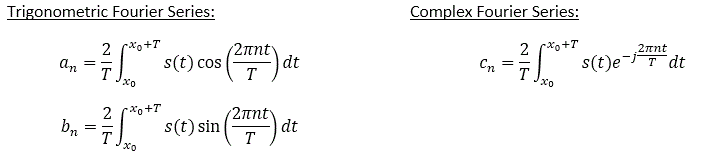Zero-index coefficients are special case:

The a0 coefficient is the equal to twice the value of the DC offset of the signal: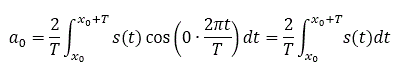The b0 coefficient is always zero: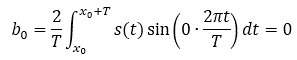Complex coefficients can be obtained from trigonometric coefficients as follows: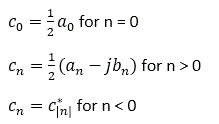Frequency domain to Time Domain

The time domain signals can be reconstructed using the following formulas: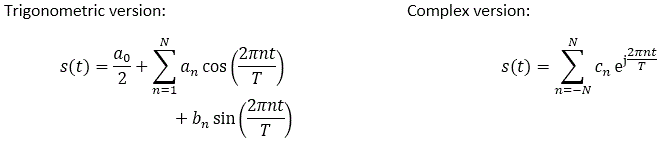The Euler identity: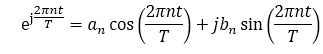Fourier Series of Square Wave

For square wave with period T and x0 = -T/2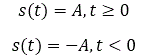Split the a[n] evaluation integral to two parts, <-T/2,0> and (0,T/2>: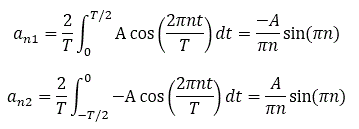Therefore: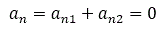Split the b[n] evaluation integral to two parts: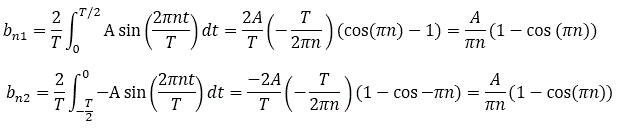Therefore: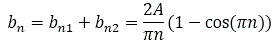The complex coefficients can be obtained from trigonometric coefficients as follows: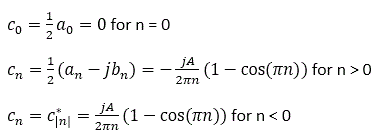Fourier Series of Full-wave Rectified Sine Wave

Utilize the symmetry and the effectively doubled frequency: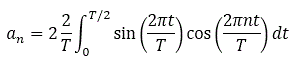Use a trig identity: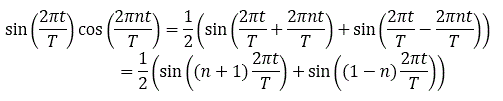Therefore: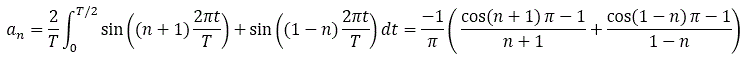Even harmonics are zero due to the cosine term on the first line. The DC component is not zero.

Further, let's calculate the b[n] coefficient: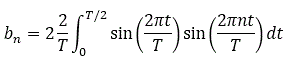Use a trig identity: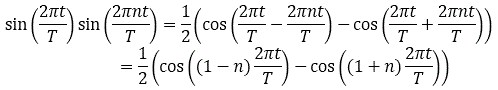Therefore: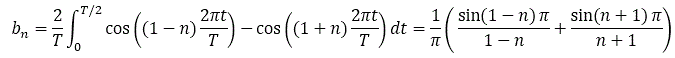All b[n] terms are zero since n is a natural number.

Time domain phase shift vs. Frequency Spectrum

As can be seen in the interactive demo above, the magnitude spectrum does not change with regards to time-domain phase shift.

The amount of time domain phase shift is directly correlated to the phase of the first harmonic.

E.g., look at the phase spectrum of these three square wave cases:

1) Zero time aligned with rising edge (non-interactive snapshot only):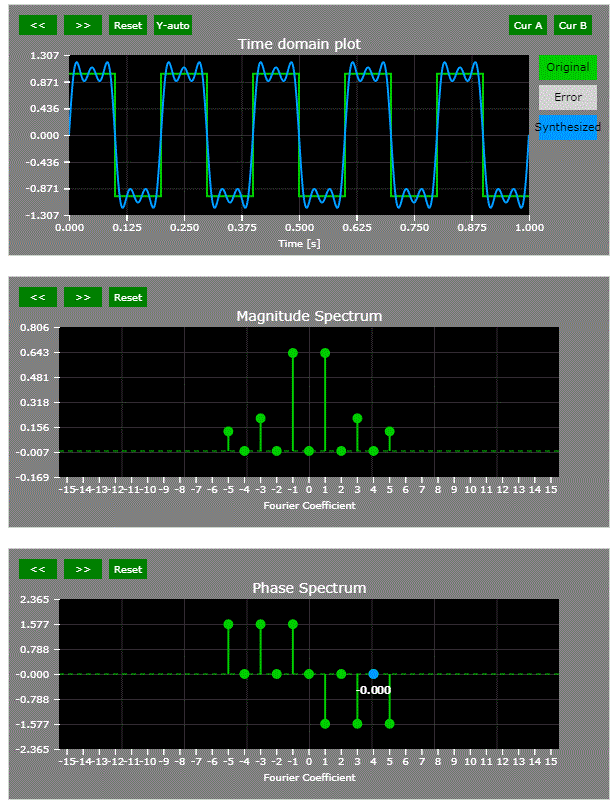2) Zero time aligned with the center of the flattop (non-interactive snapshot only):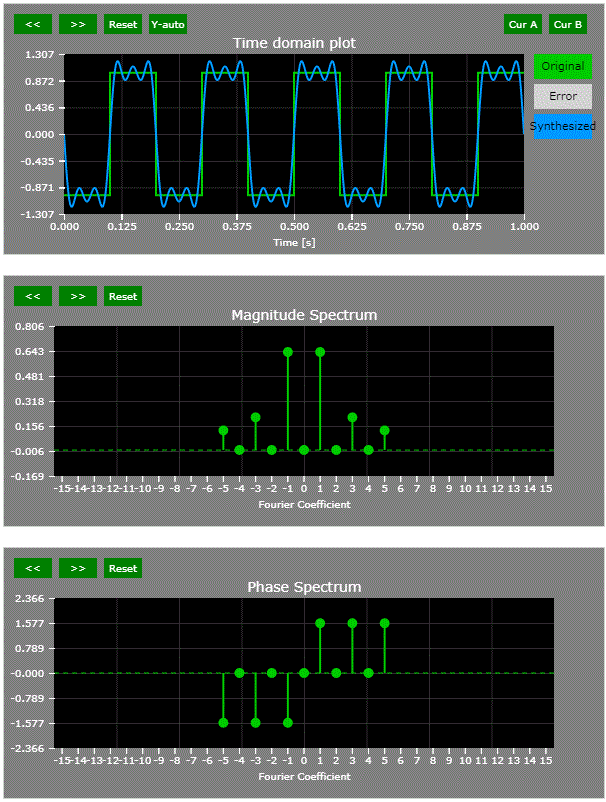3) Zero time aligned with falling edge (non-interactive snapshot only):#### Thought Nuggets

Q: If the time-domain signal is continuous, why is the frequency-domain spectrum not continuous as well?

A: Fourier series describes signals that are repetitive with period T. If a signal is not periodic, its effective period T becomes large and the Fourier Series coefficients become more dense. When T -> infinity, the Fourier Series spectrum becomes continuous and would be called Fourier Transform.